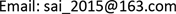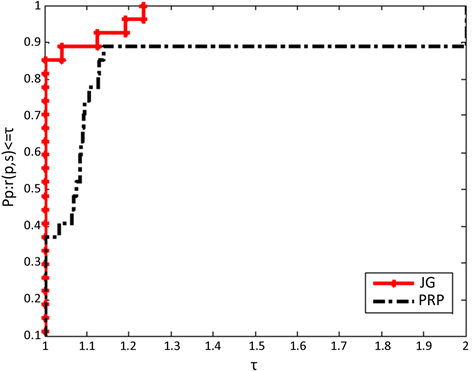﻿ 一种新的三项共轭梯度法求解非线性方程组 A New Three-Term Conjugate Gradient Method for Solving Nonlinear Equations

Advances in Applied Mathematics
Vol. 08  No. 05 ( 2019 ), Article ID: 30173 , 7 pages
10.12677/AAM.2019.85097

A New Three-Term Conjugate Gradient Method for Solving Nonlinear Equations

Ruosha Liao

College of Mathematics and Information Science, Guangxi University, Nanning GuangxiReceived: Apr. 16th, 2019; accepted: May 7th, 2019; published: May 14th, 2019ABSTRACT

Based on the existing three-term conjugate gradient method, this paper designs a new conjugate gradient method JG to solve the problem of nonlinear equations, and proves the sufficient descent and global convergence of JG algorithm under certain assumptions. From the results of numerical experiments, we can see that JG algorithm has better properties than PRP algorithm.

Keywords:Conjugate Gradient Method, Sufficient Descent Property, Global ConvergenceCopyright © 2019 by author(s) and Hans Publishers Inc.

This work is licensed under the Creative Commons Attribution International License (CC BY).

http://creativecommons.org/licenses/by/4.0/1. 引言

$h\left(x\right)=0,x\in {R}^{n}$ (1)

$\mathrm{min}F\left(x\right),x\in {R}^{n}$ (2)

${x}_{k+1}={x}_{k}+{\alpha }_{k}{d}_{k}$

$\begin{array}{l}F\left({x}_{k+1}\right)-F\left({x}_{k}\right)\le {c}_{1}{\alpha }_{k}{g}_{k}^{\text{T}}{d}_{k}\\ {g}_{k+1}^{\text{T}}{d}_{k}\ge {c}_{2}{g}_{k}^{\text{T}}{d}_{k}\end{array}$

$-g{\left({x}_{k}+{\alpha }_{k}{d}_{k}\right)}^{\text{T}}{d}_{k}\ge \sigma {\alpha }_{k}‖g\left({x}_{k}+{\alpha }_{k}{d}_{k}\right)‖{‖{d}_{k}‖}^{2}$ (3)

Zhang  提出了一种三项共轭梯度算法：

${d}_{k}=\left\{\begin{array}{l}-{g}_{k}+{\beta }_{k}^{PRP}{d}_{k-1}-{v}_{k}{y}_{k}\text{}\text{ }\text{ }\text{if}\text{\hspace{0.17em}}k\ge 1\\ -{g}_{k}\text{if}\text{\hspace{0.17em}}k=0\end{array}$

${d}_{k}^{\text{T}}{g}_{k}=-{‖{g}_{k}‖}^{2}$

${d}_{k}=\left\{\begin{array}{l}-{g}_{k}+\frac{{g}_{k}^{\text{T}}{y}_{k}^{*}{d}_{k-1}-{g}_{k}^{\text{T}}{d}_{k-1}{y}_{k}^{*}}{\mu ‖{d}_{k-1}‖‖{y}_{k}^{*}‖+v{‖{y}_{k}^{*}‖}^{2}+{‖{g}_{k-1}‖}^{2}+\eta ‖{g}_{k-1}‖‖{d}_{k-1}‖+r{‖{d}_{k-1}‖}^{2}}\text{if}\text{\hspace{0.17em}}k\ge 1\\ -{g}_{k}\text{}\text{\hspace{0.17em}}\text{\hspace{0.17em}}\text{\hspace{0.17em}}\text{\hspace{0.17em}}\text{}\text{\hspace{0.17em}}\text{}\text{ }\text{ }\text{if}\text{\hspace{0.17em}}k=0\end{array}$ (4)

2. 算法

Step 0：令初始点 ${x}_{0}\in R$$\mu >0$$v>0$$\eta >0$$r>0$$\rho ,\epsilon \in \left(0,1\right)$$k:=1$

Step 1：若 $‖g\left(x\right)‖\le \epsilon$ ，停止；否则转到Step 2；

Step 2：通过(4)式计算搜索方向 ${d}_{k}$

Step 3：选择满足条件(3)的步长 ${\alpha }_{k}$

Step 4：令迭代公式为 ${w}_{k}={x}_{k}+{\alpha }_{k}{d}_{k}$

Step 5：若 $‖g\left(x\right)‖\le \epsilon$ ，停止，令 ${x}_{k+1}={w}_{k}$ ；否则令

${x}_{k+1}={x}_{k}-\frac{g{\left({w}_{k}\right)}^{\text{T}}\left({x}_{k}-{w}_{k}\right)}{{‖g\left({w}_{k}\right)‖}^{2}}g\left({w}_{k}\right)$ (5)

Step 6：令 $k:=k+1$ ，转Step 1。

3. 充分下降性和全局收敛性

$‖g\left(x\right)-g\left(y\right)‖\le L‖x-y‖,\text{}\forall x,y\in {R}^{n}$ (6)

$‖{g}_{k}‖\le \varsigma$

${g}_{k+1}{d}_{k+1}\le -{‖{g}_{k+1}‖}^{2}$ (7)

$‖{g}_{k}‖\le ‖{d}_{k}‖\le \left(1+\frac{2}{\mu }\right)‖{g}_{k}‖$ (8)

$\begin{array}{c}‖{d}_{k}‖=‖-{g}_{k}+\frac{{g}_{k}^{\text{T}}{y}_{k}^{*}{d}_{k-1}-{g}_{k}^{\text{T}}{d}_{k-1}{y}_{k}^{*}}{\mu ‖{d}_{k-1}‖‖{y}_{k}^{*}‖+v‖{y}_{k}^{*}‖+{‖{g}_{k-1}‖}^{2}+\eta ‖{g}_{k-1}‖‖{d}_{k-1}‖+r{‖{d}_{k-1}‖}^{2}}‖\\ \le ‖-{g}_{k}‖+‖\frac{{g}_{k}^{\text{T}}{y}_{k}^{*}{d}_{k-1}-{g}_{k}^{\text{T}}{d}_{k-1}{y}_{k}^{*}}{\mu ‖{d}_{k-1}‖‖{y}_{k}^{*}‖+v‖{y}_{k}^{*}‖+{‖{g}_{k-1}‖}^{2}+\eta ‖{g}_{k-1}‖‖{d}_{k-1}‖+r{‖{d}_{k-1}‖}^{2}}‖\\ \le ‖-{g}_{k}‖+\frac{2‖{g}_{k}^{\text{T}}‖‖{y}_{k}^{*}‖‖{d}_{k-1}‖}{\mu ‖{d}_{k-1}‖‖{y}_{k}^{*}‖}=\left(1+\frac{2}{\mu }\right)‖{g}_{k}‖\end{array}$

${\alpha }_{k}\ge \mathrm{min}\left\{s,\frac{\rho }{L+\sigma ‖g\left({\stackrel{^}{w}}_{k}\right)‖}\frac{{‖g\left({x}_{k}\right)‖}^{2}}{{‖{d}_{k}‖}^{2}}\right\}$

$-g{\left({x}_{k}+{\alpha }_{k}{d}_{k}\right)}^{\text{T}}{d}_{k}<\sigma {\alpha }_{k}‖g\left({x}_{k}+{\alpha }_{k}{d}_{k}\right)‖{‖{d}_{k}‖}^{2}$

${‖{g}_{k}‖}^{2}\le -{g}_{k}^{\text{T}}{d}_{k}\le {\left(g\left({\stackrel{^}{w}}_{k}\right)-g\left({x}_{k}\right)\right)}^{\text{T}}{d}_{k}+\sigma {\stackrel{^}{\alpha }}_{k}‖g\left({\stackrel{^}{w}}_{k}\right)‖{‖{d}_{k}‖}^{2}\le {\stackrel{^}{\alpha }}_{k}\left(L+\sigma ‖g\left({\stackrel{^}{w}}_{k}\right)‖\right){‖{d}_{k}‖}^{2}$

${\alpha }_{k}\ge \frac{\rho }{L+\sigma ‖g\left({\stackrel{^}{w}}_{k}\right)‖}\frac{{‖g\left({x}_{k}\right)‖}^{2}}{{‖{d}_{k}‖}^{2}}$

${‖{x}_{k+1}-{x}_{*}‖}^{2}\le {‖{x}_{k}-{x}_{*}‖}^{2}-{‖{x}_{k+1}-{x}_{k}‖}^{2}$ (9)

$\underset{k=0}{\overset{\infty }{\sum }}{‖{x}_{k+1}-{x}_{k}‖}^{2}<\infty$ (10)

$〈g\left({w}_{k}\right)-g\left({x}_{*}\right),{x}_{*}-{w}_{k}〉=〈g\left({w}_{k}\right),{x}_{*}-{w}_{k}〉\le 0$

${x}_{k+1}$${x}_{k}$${M}_{k}=\left\{x\in {R}^{n}|〈g\left({w}_{k}\right),x-{w}_{k}〉\le 0\right\}$ 上的投影。如果 ${x}_{*}$${M}_{k}$ 上，则 $〈{x}_{k}-{x}_{k+1},{x}_{k+1}-{x}_{*}〉\ge 0$ 。可得：

${‖{x}_{k}-{x}_{*}‖}^{2}={‖{x}_{k}-{x}_{k+1}‖}^{2}+{‖{x}_{k+1}-{x}_{*}‖}^{2}+2〈{x}_{k}-{x}_{k+1},{x}_{k+1}-{x}_{*}〉\ge {‖{x}_{k}-{x}_{k+1}‖}^{2}+{‖{x}_{k+1}-{x}_{*}‖}^{2}$

${‖{x}_{k+1}-{x}_{k}‖}^{2}\le {‖{x}_{k}-{x}_{*}‖}^{2}-{‖{x}_{k+1}-{x}_{*}‖}^{2}$

$‖{x}_{k+1}-{x}_{k}‖=\frac{|g{\left({w}_{k}\right)}^{\text{T}}\left({x}_{k}-{w}_{k}\right)|}{‖g\left({w}_{k}\right)‖}=\frac{-{\alpha }_{k}g{\left({w}_{k}\right)}^{\text{T}}{d}_{k}}{‖g\left({w}_{k}\right)‖}\ge \sigma {\alpha }_{k}^{2}{‖{d}_{k}‖}^{2}$

$‖{x}_{k+1}-{x}_{k}‖\to 0$

$\underset{k\to +\infty }{\mathrm{lim}}{\alpha }_{k}{d}_{k}=0$ (11)

$\underset{k\to \infty }{\mathrm{lim}}\mathrm{inf}‖{g}_{k}‖=0$ (12)

${c}_{3}\delta \le {c}_{3}‖{g}_{k}‖\le ‖{d}_{k}‖\le {c}_{4}‖{g}_{k}‖\le {c}_{4}\zeta$

$\begin{array}{c}{\alpha }_{k}‖{d}_{k}‖\ge \mathrm{min}\left\{s,\frac{\rho }{L+\sigma ‖g\left({\stackrel{^}{w}}_{k}\right)‖}\frac{{‖g\left({x}_{k}\right)‖}^{2}}{{‖{d}_{k}‖}^{2}}\right\}‖{d}_{k}‖\\ \ge \mathrm{min}\left\{{c}_{3}\delta s,\frac{\rho \delta }{{c}_{4}\zeta \left(L+\sigma \varsigma \right)}\right\}>0\end{array}$

4. 数值实验Figure 1. Algorithm JG and algorithm PRP performance chart (CT)

5. 结论

A New Three-Term Conjugate Gradient Method for Solving Nonlinear Equations[J]. 应用数学进展, 2019, 08(05): 869-875. https://doi.org/10.12677/AAM.2019.85097

1. 1. Polyak, B.T. (1969) The Conjugate Gradient Method in Extremal Problems. Ussr Computational Mathematics & Mathematical Physics, 9, 94-112. https://doi.org/10.1016/0041-5553(69)90035-4

2. 2. Dai, Y. and Yuan, Y. (1999) A Nonlinear Conjugate Gradient Method with a Strong Global Convergence Property. Siam Journal on Optimization, 10, 177-182. https://doi.org/10.1137/S1052623497318992

3. 3. Fletcher, R. and Reeves, C.M. (1964) Function Minimization by Conjugate Gradients. Computer Journal, 7, 149-154. https://doi.org/10.1093/comjnl/7.2.149

4. 4. Polak, E. and Ribiere, G. (1968) Note sur la convergence de methodes de directions conjuguees. Revue Française D’-informatique et de Recherche Opérationnelle, 16, 35-43. https://doi.org/10.1051/m2an/196903R100351

5. 5. Wei, Z., Yao, S. and Liu, L. (2006) The Convergence Prop-erties of Some New Conjugate Gradient Methods. Applied Mathematics & Computation, 183, 1341-1350. https://doi.org/10.1016/j.amc.2006.05.150

6. 6. Yuan, G. and Lu, X. (2008) A New Backtracking Inexact BFGS Method for Symmetric Nonlinear Equations. Computers & Mathematics with Applications, 55, 116-129. https://doi.org/10.1016/j.camwa.2006.12.081

7. 7. Zhang, L., Zhou, W. and Li, D.H. (2006) A Descent Modified Polak-Ribiere-Polyak Conjugate Gradient Method and Its Global Convergence. IMA Journal of Numerical Analysis, 26, 629-640. https://doi.org/10.1093/imanum/drl016

8. 8. Solodov, M.V. and Svaiter, B.F. (1998) A Globally Con-vergent Inexact Newton Method for Systems of Monotone Equations. Reformulation: Nonsmooth, Piecewise Smooth, Semismooth and Smoothing Methods. Springer, US, 355-369. https://doi.org/10.1007/978-1-4757-6388-1_18

9. 9. Yuan, G., Wei, Z. and Lu, X. (2011) A BFGS Trust-Region Method for Nonlinear Equations. Computing, 92, 317-333. https://doi.org/10.1007/s00607-011-0146-z